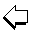ULIB User Group for Laser Interference BiometryThe following rules of thumb may be applied as a starting point to estimate new IOL constants for optical biometry with the Zeiss IOLMaster:

 (1) AIOLMaster = AUltrasound + 3 * (ALIOLMaster - ALUltrasound) where A IOLMaster : (optimized) A-constant for optical biometry (with the IOLMaster) A Ultrasound : (optimized) A-constant for acoustical biometry (ultrasound a-scan and keratometer) ALIOLMaster : mean of axial length as measured with the IOLMaster ALUltrasound : mean of axial length as measured by ultrasound e.g. 119.6 = 119.3 + 3 * (22.98 - 22.88)

For formula (1) it is assumed that keratometry results with the IOLMaster and the hitherto used separate keratometer are identical. If this is not the case, i.e. if there is a difference in the mean corneal radii measured with the two instruments, formula (2) applies:

 (2) AIOLMaster = AUltrasound + 3 * (ALIOLMaster - ALUltrasound) - 6 * (RIOLMaster - RKeratometer) where A IOLMaster : (optimized) A-constant for optical biometry (with the IOLMaster) A Ultrasound : (optimized) A-constant for acoustical biometry (ultrasound a-scan and keratometer) ALIOLMaster : mean of axial length as measured with the IOLMaster ALUltrasound : mean of axial length as measured by ultrasound RIOLMaster : mean of corneal radii as measured with the IOLMaster RKeratometer : mean of corneal radii as measured by separate keratometer e.g. 119.6 = 119.6 + 3 * (22.98 - 22.88) - 6 * (7.80-7.75)ULIB Home